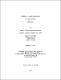## Thermal plasma processing of fine grained materials2012
##### Publisher
University of Alabama Libraries
##### Abstract

Synthesis of advanced ceramic materials has been systematically investigated using non-transferred thermal plasma reactor. Low cost oxide feed materials has been used as the solid feed to the reactor while methane (CH_4 ) and argon (Ar) were used as reducing and carrier gases, respectively. A 2D computational fluid dynamics (CFD) based mathematical model was developed to understand the flow and temperature profiles inside the reactor. The concept was extended to a 3D model and a comparison was made with the results from 2D model. The velocity increases linearly with increase in the pressure in both the 2D and the 3D models while the maximum velocity from 3D model is lower by about 35-40 m/s at any given pressure. A decrease in the residence time was observed in the 3D model compared to the 2D model. An intermediate plasma gas pressure of 45 psi was used in experiments to ensure high temperatures and residence times in the reactor. Thermochemical calculations have been carried out to determine the molar ratio of the reducing gas to be used in TiO_2 -B_2 O_3 -CH_4 and SiO_2 -CH_4 system. The maximum theoretical yield of TiB_2 of about 82 mol% was obtained at a molar ratio of TiO_2 :B_2 O_3 :CH_4 = 1:1:5. Maximum theoretical yield of SiC of about 97 mol% was obtained at a molar ratio of SiO_2 :CH_4 =1:3 at a temperature of 1520ºC. Experiments were carried out using thermal plasma reactor to synthesize TiB_2 and SiC. The maximum yield of TiB_2 of about 40 mol% was obtained with a feed molar ratio of TiO_2 :B_2 O_3 :CH_4 = 1:1:5 and a power of 23.4 kW. Relatively higher solid feed rates increased the yield of TiB_2 . The TiB_2 spherical particles formed are in the range of 20-100 nm. A change in crystal structure was observed in TiO_2 from anatase to rutile. Experiments using a molar ratio of SiO_2 :CH_4 = 1:2 produced maximum yield of SiC of about 65 mol% at a solid feed rate of 5 g/min. Mostly spherical morphology with some nanorods have been observed. The presence of Si had been observed and was quantified using XRD, HR-TEM, Raman and XPS.

##### Description
Electronic Thesis or Dissertation
##### Keywords
Materials science, Nanotechnology